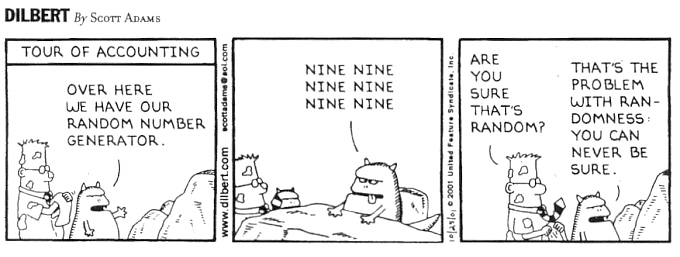# Shuffle a list (with duplicates) to avoid identical elements being next to each other

I am wondering if there is a "best" way to shuffle a list of elements that contains duplicates such that the case where array[i] == array[i+1] is avoided as much as possible.

I am working on a weighted advertising display (I can adjust the number of displays per rotation for any given advertiser) and would like to avoid the same advertister appearing twice in a row.

This is pretty similar to this question. If you replace A, B, and C in the example given over there with your advertisers, I think you arrive at the same problem. Maybe some of the solutions suggested for that one can help you.

Basic randomizing should cause enough dispersion in a large set.

If you want to minimize that even more (which might not even be necessary depending on the sets), the simplest way would be to definitely find the close by dupes after randomization and move them around (but you might create patterns). A better approach might be to create subsets containing the side by side dupes and redo the randomization.

For a smaller set nothing might be possible, depending on the number of dupes. So the solution for a very small set would only be good basic randomization (And we're back at the first sentence).Personally I think the easiest way to hand this would be to randomize the array, and then iterate over it until you find 2 elements with the same value that are next to each other. When you find 2 of the same values beside eachother, move the later one to another spot in the array by iterating over the array until you find a spot such that it isn't beside another of the same value. If you can't find a value, just leave it where it is, and continue on with the next element of the array. This probably won't be the most optimal solution, but will be fine for smaller data sets, and probably the simplest to program.

• hmm, could be okay but that's what up to O(n^2) ? Dec 11, 2008 at 2:57
• Probably about O(n^2), but the average case would probable be a lot lower if you don't expect a lot of duplicates. Dec 11, 2008 at 3:00
• Yeah, and the algorithm is straight-forward at least - can always optimise later. Wish they'd taught us shuffle problems at uni though. Dec 11, 2008 at 3:01
• The very worst case would be O(n^2), but I wouldn't expect you to get there with a random shuffle. Your average case is going to be close to O(n). Dec 11, 2008 at 3:02
• Jeff has a good post on shuffling here -> codinghorror.com/blog/archives/001008.html Dec 11, 2008 at 3:03

What's the biggest number of duplicates you may have? 2, 3, any?

• Well because of the weighting e.g. advertiser 1 could appear 5 times per rotation there could be 5 duplicates easily. Dec 11, 2008 at 2:57

For reference, my (very) naive approach was something like this (actually using LINQ/SQL calls but this is simplified):

``````var advertisers = getAdvertisers();
var returnList = new List();
int totalWeight = sumOfAllAdvertisersWeight();
while (totalWeight > 0)
{
for (int i=0; i<advertisers.Count; i++)
{
if (advertisers[i].Weight > 0)
{